# 3.3 Designing Data Types

In this section we focus on developing APIs as a critical step in the development of any program. We need to consider various alternatives, understand their impact on both client programs and implementations, and refine the design to strike an appropriate balance between the needs of clients and the possible implementation strategies.

## Designing APIs

In Section 3.1, we composed client programs that use APIs; in Section 3.2, we implemented APIs. Now we consider the challenge of designing APIs.

### Standards.

It is easy to understand why conforming to an API is so important by considering other domains. From railroad tracks, to threaded nuts and bolts, to MP3s and DVDs, to radio frequencies, to Internet standards, we know that using a common standard interface enables the broadest usage of a technology. By using APIs to separate clients from implementations, we reap the benefits of standard interfaces for every program that we compose.

### Specification problem.

Our APIs for data types are sets of methods, along with brief English-language descriptions of what the methods are supposed to do. Ideally, an API would clearly articulate behavior for all possible arguments, including side effects, and then we would have software to check that implementations meet the specification. Unfortunately, a fundamental result from theoretical computer science, known as the specification problem, says that this goal is actually impossible to achieve. Therefore, we resort to informal descriptions with examples, such as those in the text surrounding our APIs.

### Wide interfaces.

A wide interface is one that has an excessive number of methods. An important principle to follow in designing an API is to avoid wide interfaces.

One of the primary purposes of developing a data type is to simplify client code. Therefore, it makes sense to pay attention to client code from the start when designing an API. Composing two clients is even better. Starting with client code is one way of ensuring that developing an implementation will be worth the effort.

### Avoid dependence on representation.

Usually when developing an API, we have a representation in mind. After all, a data type is a set of values and a set of operations defined on those values, and it does not make much sense to talk about the operations without knowing the values. But that is different from knowing the representation of the values. One purpose of the data type is to simplify client code by allowing it to avoid details of and dependence on a particular representation.

### Pitfalls in API design.

An API may be too hard to implement, implying implementations that are difficult or impossible to develop, or too hard to use, creating client code that is more complicated than without the API. An API might be too narrow, omitting methods that clients need, or too wide, including a large number of methods not needed by any client. An API may be too general, providing no useful abstractions, or too specific, providing abstractions so detailed or so diffuse as to be useless. These considerations are sometimes summarized in the motto: provide to clients the methods they need and no others.

## Encapsulation

The process of separating clients from implementations by hiding information is known as encapsulation. Details of the implementation are kept hidden from clients, and implementations have no way of knowing details of client code, which may even be created in the future. We use encapsulation to enable modular programming, facilitate debugging, and clarify program code. These reasons are tied together (well-designed modular code is easier to debug and understand than code based entirely on built-in types).

### Modular programming.

The key to success in modular programming is to maintain independence among modules. We do so by insisting on the API being the only point of dependence between client and implementation — data-type implementation code can assume that the client knows nothing but the API.

### Changing an API.

We often reap the benefits of encapsulation when we use standard modules. New versions of Python often, for example, may include new implementations of various data types or modules that define functions. There is a strong and constant motivation to improve data-type implementations because all clients can potentially benefit from an improved implementation. However, Python APIs rarely change. When changes do occur, they are costly throughout the Python community — everyone has to update their clients. So once a significant number of clients are using a module, try not to change its API.

### Changing an implementation.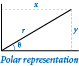Consider the class `Complex`, defined in complexpolar.py. It has the same name and API as the class `Complex` defined in complex.py (from Section 3.2), but uses a different representation for the complex numbers. The `Complex` class defined in complex.py uses the Cartesian representation, where instance variables `_re` and `_im` represent a complex number as x + yi. The `Complex` class defined in complexpolar.py uses the polar representation, where instance variables `_r` and `_theta` represent complex numbers as r(cos θ + i sin θ). In this representation, we refer to r as the magnitude and θ as the polar angle. The polar representation is of interest because certain operations on complex numbers are easier to perform in the polar representation. Addition and subtraction are easier in the Cartesian representation; multiplication and division are easier in the polar representation. The idea of encapsulation is that we can substitute one of these programs for the other (for whatever reason) without changing client code, except to change the `import` statement to use `complexpolar` instead of `complex`.

### Private.

Many programming languages provide support for enforcing encapsulation. For example, Java provides the `private` visibility modifier. When you declare an instance variable (or method) to be private, you are making it impossible for any client (code in another module) to directly access the instance variable (or method) that is the subject of the modifier. Python does not offer a `private` visibility modifier, which means that clients can directly access all instance variables, methods, and functions. However the Python programming community espouses a pertinent convention: if an instance variable, method, or function has a name that begins with an underscore, then clients should consider that instance variable, method, or function to be private. Through this naming convention, clients are informed that they should not directly access the instance variable, method, or function thus named.

In this booksite we always make all instance variables private in our classes. We strongly recommend that you do the same — there is no good reason to access an instance variable directly from a client.

### Limiting the potential for error.

Encapsulation also helps programmers ensure that their code operates as intended. For example, in the 2000 presidential election, Al Gore received negative 16,022 votes on an electronic voting machine in Volusia County, Florida. The counter variable was not properly encapsulated in the voting machine software! To understand the problem, consider counter.py, which defines a simple `Counter` class according to this API: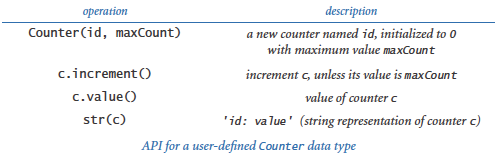This abstraction is useful in many contexts, including, for example, an electronic voting machine. It encapsulates a single integer and ensures that the only operation that can be performed on the integer is increment by one. Therefore, it can never go negative. Proper encapsulation is far from a complete solution to the voting security problem, but it is a good start.

### Code clarity.

Precisely specifying a data type improves design because it leads to client code that can more clearly express its computation. You have seen many examples of such client code in Sections 3.1 and 3.2, from charged particles to pictures to complex numbers. One key to good design is to observe that code composed with the proper abstractions can be nearly self-documenting.

## Immutability

An object from a data type is immutable if its data-type value cannot change once created. An immutable data type, such as a Python string, is one in which all objects of that type are immutable. By contrast, a mutable data type, such as a Python list/array, is one in which objects of that type have values that are designed to change. Of the data types considered in this chapter, `Charge`, `Color`, and `Complex` are all immutable, and `Picture`, `Histogram`, `Turtle`, `StockAccount`, and `Counter` are all mutable. Whether to make a data type immutable is a fundamental design decision and depends on the application at hand.

### Immutable data types.

The purpose of many data types is to encapsulate values that do not change. For example, a programmer implementing a `Complex` client might reasonably expect to compose the code `z = z0`, thus setting two variables to reference the same `Complex` object, in the same way as for floats or integers. But if `Complex` were mutable and the object referenced by `z` were to change after the assignment `z = z0`, then the object referenced by `z0` would also change (they are aliases, or both references to the same object). Conceptually, changing the value of `z` would change the value of `z0`! This unexpected result, known as an aliasing bug, comes as a surprise to many newcomers to object-oriented programming.

### Mutable data types.

For many other data types, the very purpose of the abstraction is to encapsulate values as they change. The `Turtle` class defined in turtle.py (from Section 3.2) is a prime example. Similarly, `Picture`, `Histogram`, `StockAccount`, `Counter`, and Python lists/arrays are all types where we expect values to change.

### Arrays and strings.

You have already encountered this distinction as a client programmer, when using Python lists/arrays (mutable) and Python strings (immutable). When you pass a string to a method/function, you do not need to worry about that method/function changing the sequence of characters in the string. In contrast, when you pass an array to a method/function, the method/function is free to change the elements of the array. Python strings are immutable because we generally do not want `str` values to change; Python arrays are mutable because we often do want array elements to change.

### Advantages of immutability.

Generally, immutable data types are easier to use and harder to misuse because the scope of code that can change object values is far smaller than for mutable types.

### Cost of immutability.

The downside of immutability is that you must create a new object for every value. For example, when you are using the `Complex` data type, the expression `z = z*z + z0` involves creating a third object (to hold the value `z*z`), then using that object with the `+` operator (without saving an explicit reference to it) and creating a fourth object to hold the value `z*z + z0`, and assigning that object to `z` (thereby orphaning the original reference to `z`). A program such as mandelbrot.py (from Section 3.2) creates a huge number of such intermediate objects. However, this expense is normally manageable because Python's memory management is typically optimized for such situations.

### Defensive copies.

Suppose that we wish to develop an immutable data type named `Vector`, whose constructor takes an array of floats as an argument to initialize an instance variable. Consider this attempt:

 ```class Vector: def __init__(self, a): self._coords = a # array of coordinates ... ```

This code makes `Vector` a mutable data type. A client program could create a `Vector` object by specifying the elements in an array, and then (bypassing the API) change the elements of the Vector after creation:

 ```a = [3.0, 4.0] v = Vector(a) a = 17.0 # bypasses the public API ```

To ensure immutability of a data type that includes an instance variable of a mutable type, the implementation needs to make a local copy, known as a defensive copy. Recall from Section 1.4 that the expression `a[:]` creates a copy of array `a[]`. As a consequence, this code creates a defensive copy:

 ```class Vector: def __init__(self, a): self._coords = a[:] # array of coordinates ... ```

Next, we consider a full implementation of such a data type.

## Example: Spatial vectors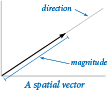A spatial vector is an abstract entity that has a magnitude and a direction. Spatial vectors provide a natural way to describe properties of the physical world, such as force, velocity, momentum, or acceleration. One standard way to specify a vector is as an arrow from the origin to a point in a Cartesian coordinate system: the direction is the ray from the origin to the point and the magnitude is the length of the arrow (distance from the origin to the point). To specify the vector, it suffices to specify the point.

This concept extends to any number of dimensions: an ordered list of n real numbers (the coordinates of an n-dimensional point) suffices to specify a vector in n-dimensional space. By convention, we use a boldface letter to refer to a vector and numbers or indexed variable names (the same letter in italics) separated by commas within parentheses to denote its value. For example, we might use x to denote the vector (x0, x1, ..., xn-1) and y to denote the vector (y0, y1, ..., yn-1).

### API.

The basic operations on vectors are to add two vectors, multiply a vector by a scalar (a real number), compute the dot product of two vectors, and compute the magnitude and direction, as follows:

• Addition: x + y = (x0 + y0, x1 + y1, ..., xn-1 + yn-1)
• Scalar product: αx = (αx0, αx1, ..., αxn-1)
• Dot product: x · y = x0y0 + x1y1 + ... + xn-1yn-1
• Magnitude: |x| = (x02 + x12 + ... + xn-12)1/2
• Direction: x / |x| = (x0 / |x|, x1 / |x|, ..., xn-1 / |x|)

Those definitions lead to this API: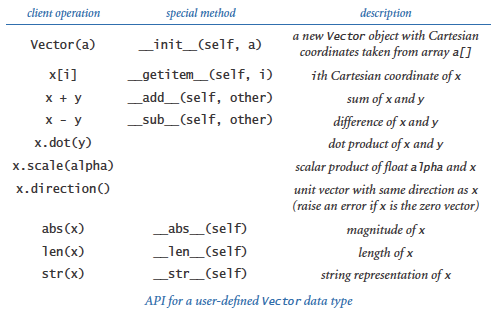As with `Complex`, this API does not explicitly specify that the data type is immutable, but we know that client programmers (who are likely to be thinking in terms of the mathematical abstraction) will certainly expect that convention, and perhaps we would rather not explain to them that we are trying to protect them from aliasing bugs!

### Representation.

Our first choice in developing an implementation is to choose a representation for the data. Using an array to hold the Cartesian coordinates provided in the constructor is a clear choice, but not the only reasonable choice. If warranted, the implementation can change the coordinate system without affecting client code.

### Implementation.

Given the representation, the code that implements all of these operations is straightforward, as you can see in the `Vector` class defined in vector.py. The constructor makes a defensive copy of the client array and none of the methods assigns a value to the copy, so that `Vector` objects are immutable.

How can we ensure immutability when it seems that the client is free to compose code like `x[i] = 2.0`? The answer to this question lies in a special method that we do not implement in an immutable data type: in such a case, Python calls the special method `__setitem__()` instead of `__getitem__()`. Since `Vector` does not implement that method, such client code would raise an `AttributeError` at run time.

## Tuples

Python's built-in `tuple` data type represents an immutable sequence of objects. It is similar to the built-in `list` data type (which we use for arrays), except that once you create a tuple, you cannot change its items. You can manipulate tuples using familiar array notation, as documented in this API: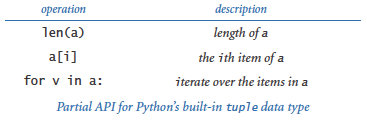You can create tuples either using the built-in function tuple() or by listing a sequence of expressions, separated by commas, and (optionally) enclosed in matching parentheses.

Using tuples can improve the design of a program. For example, if we replace the first statement in the constructor of vector.py with

 ```self._coords = tuple(a) ```

then any attempt to change a vector coordinate within the Vector class raises a `TypeError` at run time, helping to enforce immutability of `Vector` objects.

Python also provides a powerful tuple assignment feature known as tuple packing and tuple unpacking that lets you assign a tuple of expressions on the right-hand side of an assignment operator to a tuple of variables on the left-hand side (provided the number of variables on the left matches the number of expressions on the right). You can use this feature to assign multiple variables simultaneously. For example, the following statement exchanges the object references in variable `x` and `y`:

 ```x, y = y, x ```

You can also use tuple packing and unpacking to return multiple values from a function (see an exercise at the end of this section).

## Polymorphism

Often, when we compose methods (or functions), we intend for them to work only with objects of specific types. Sometimes, we want them to work with objects of different types. A method (or function) that can take arguments with different types is said to be polymorphic.

The best kind of polymorphism is the unexpected kind: when you apply an existing method/function to a new data type (for which you never planned) and discover that the method/function has exactly the behavior that you wanted. The worst kind of polymorphism is also the unexpected kind: when you apply an existing method/function to a new data type and it returns the wrong answer! Finding a bug of this sort can be an extraordinary challenge.

### Duck typing.

Duck typing is a programming style in which the language does not formally specify the requirements for a function's arguments; instead, it just tries to call the function if a compatible one is defined (and raises a run-time error otherwise). The name comes from an old quote attributed to the poet J. W. Riley:

When I see a bird that
walks like a duck and swims like a duck and quacks like a duck
I call that bird a duck

In Python, if an object walks like a duck, swims like a duck, and quacks like a duck, you can treat that object as a duck; you don't need to explicitly declare it to be a duck. In many languages (such as Java or C++), you do need to explicitly declare the types of variables, but not in Python — Python uses duck typing for all operations (function calls, method calls, and operators). It raises a `TypeError` at run time if an operation cannot be applied to an object because it is of an inappropriate type. This approach leads to simpler and more flexible client code and puts the focus on operations that are actually used rather than the type.

### Disadvantages of duck typing.

The primary disadvantage of duck typing is that it is difficult to know precisely what the contract is between the client and the implementation, especially when a required method is needed only indirectly. The API simply does not carry this kind of information. This lack of information can lead to run-time errors. Worse, the end result can be semantically incorrect, with no error raised at all. Next, we consider a simple example of this situation.

### A case in point.

We designed our `Vector` data type under the implicit assumption that the vector components would be floats and that the client would create a new vector by passing an array of `float` objects to the constructor. If the client creates two vectors `x` and `y` in this way, then both `x[i]` and `x.dot(y)` return floats and both `x + y` and `x - y` return vectors with float components, as expected.

Suppose, instead, that a client creates a `Vector` with integer components by passing an array of `int` objects to the constructor. If the client creates two vectors `x` and `y` in this manner, then both `x[i]` and `x.dot(y)` return integers and both `x + y` and `x - y` return vectors with integer components, as desired. Of course, `abs(x)` returns a `float` and `x.direction()` returns a vector with `float` components. This is the best kind of polymorphism, where duck typing works serendipitously.

Now, suppose that a client creates a `Vector` with complex components by passing an array of `complex` objects to the constructor. There is no problem with vector addition or scalar multiplication, but the implementation of the dot product operation (along with the implementations of magnitude and direction, which depend on the dot product) fails spectacularly. Here is an example:

 ```a = [1 + 2j, 2 + 0j, 4 + 0j] x = Vector(a) b = abs(x) ```

This code results in a `TypeError` at run time, with `math.sqrt()` trying to take the square root of a complex number. The problem is that the dot product of two complex-valued vectors x and y requires taking the complex conjugate of the elements in the second vector. The textbook describes the problem and its solution in detail.

In this case, duck typing is the worst kind of polymorphism. It is certainly reasonable for a client to expect the implementation of `Vector` to work properly when vector components are complex numbers. How can an implementation anticipate and prepare for all potential uses of a data type? This situation presents a design challenge that is impossible to meet. All we can do is caution you to check, if possible, that any data type that you use can handle the types of data that you intend to use with it.

The ability to define a data type that provides its own definitions of operators is a form of polymorphism known as operator overloading. In Python, you can overload almost every operator, including operators for arithmetic, comparisons, indexing, and slicing. You can also overload built-in functions, including absolute value, length, hashing, and type conversion. Overloading operators and built-in functions makes user-defined types behave more like built-in types.

To perform an operation, Python internally converts the expression into a call on the corresponding special method; to call a built-in function, Python internally calls the corresponding special method instead. To overload an operator or built-in function, you include an implementation of the corresponding special method with your own code.

### Arithmetic operators.

Python associates a special method with each of its arithmetic operators, so you can overload any arithmetic operation by implementing the corresponding special method, as detailed in this table.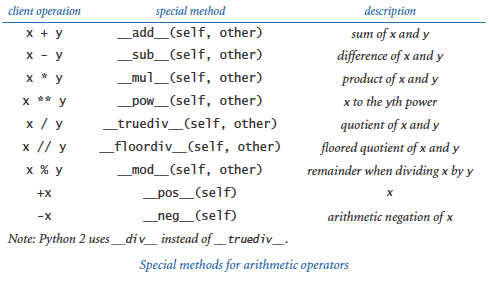### Equality.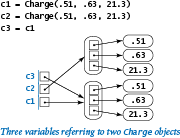The `==` and `!=` operators for testing equality require special attention. For example, consider the code in the diagram at right, which creates two `Charge` objects, referenced by three variables `c1`, `c2`, and `c3`. As illustrated in the diagram, `c1` and `c3` both reference the same object, which is different from the one referenced by `c2`. Clearly, `c1 == c3` is `True`, but what about `c1 == c2`? The answer to this question is unclear because there are two ways to think about equality in Python:

Reference equality (identity equality). Reference equality holds when two references are equal — they refer to the same object. The built in function `id()` gives the identity of an object (its memory address); the `is` and `is not` operators test whether two variables refer to the same object. That is, the implementation of `c1 is c2` tests whether `id(c1)` and `id(c2)` are the same. In our example, `c1 is c3` is `True` as expected, but `c1 is c2` is `False` because `c1` and `c2` reside at different memory addresses.

Object equality (value equality). Object equality holds when two objects are equal — they have the same data-type value. You should use the `==` and `!=` operators, defined using the special methods `__eq__()` and `__ne__()`, to test for object equality. If you do not define an `__eq__()` method, then Python substitutes the `is` operator. That is, by default `==` implements reference equality. So, in our earlier example, `c1 == c2` is `False` even though `c1` and `c2` have the same position and charge value. If we want two charges with identical position and charge value to be considered equal, we can ensure this outcome by including the following code in charge.py (from Section 3.2):

 ``` def __eq__(self, other): if self._rx != other._rx: return False if self._ry != other._ry: return False if self._q != other._q: return False return True def __ne__(self, other): return not __eq__(self, other) ```

With this code in place, `c1 == c2` is now True in our example.

### Hashing.

We now consider a fundamental operation related to equality testing, known as hashing, that maps an object to an integer, known as a hash code. This operation is so important that it is handled by Python's special method `__hash__()` in support of the built-in `hash()` function. We refer to an object as `hashable` if it satisfies the following three properties:

• The object can be compared for equality with other objects via the == operator.
• Whenever two objects compare as equal, they have the same hash code.
• The object's hash code does not change during its lifetime.

In typical applications, we use the hash code to map an object `x` to an integer in a small range, say between 0 and `m`-1, using the hash function

 ```hash(x) % m ```

Then, we can use the hash function value as an integer index into an array of length `m` (see the sketch.py program described later in this section and the hashst.py program described in Section 4.4). All of Python's immutable data types (including `int`, `float`, `str`, and `tuple`) are hashable and engineered to distribute the objects in a reasonable manner.

You can make a user-defined data type hashable by implementing the two special methods `__hash__()` and `__eq__()`. Crafting a good hash function requires a deft combination of science and engineering, and is beyond the scope of this booksite. Instead, we describe a simple recipe for doing so in Python that is effective in a wide variety of situations:

• Ensure that the data type is immutable.
• Implement `__eq__()` by comparing all significant instance variables.
• Implement `__hash__()` by putting the same instance variables into a tuple and calling the built-in `hash()` function on the tuple.

For example, following is a `__hash__()` implementation for the `Charge` data type (defined in charge.py from Section 3.2) to accompany the `__eq__()` implementation that we just considered:

 ```def __hash__(self): a = (self._rx, self._ry, self._q) return hash(a) ```

### Comparison operators.

Similarly, comparisons like `x < y` and `x >= y` are not just for integers, floats, and strings in Python. Again, Python associates a special method with each of its comparison operators, so you can overload any comparison operator by implementing the corresponding special method, as detailed in this table:As a matter of style, if you define any one of the comparison methods, then you should define all of them, and in a consistent manner. You can make a user-defined type comparable by implementing the six special methods, as we do here for the `Counter` class defined in counter.py:

 ```def __lt__(self, other): return self._count < other._count def __le__(self, other): return self._count <= other._count def __eq__(self, other): return self._count == other._count def __ne__(self, other): return self._count != other._count def __gt__(self, other): return self._count > other._count def __ge__(self, other): return self._count >= other._count ```

### Other operators.

Almost every operator in Python can be overloaded. If you want to overload an operator, you can track down the corresponding special method in the official Python documentation.

### Built-in functions.

We have been overloading the built-in function `str()` in every class we develop, and there are several other built-in functions that we can overload in the same way. The ones that we use in this book are summarized in the table below. We have already used all of these functions, except for `iter()`, which we defer until Section 4.4.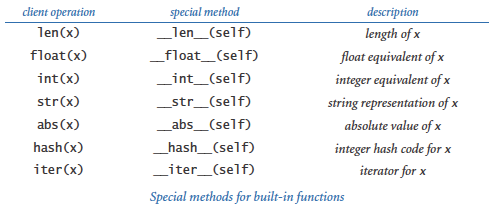## Functions are Objects

In Python, everything is an object, including functions. This means that you can use functions as arguments to functions and return them as results. Defining so-called higher-order functions that manipulate other functions is common both in mathematics and in scientific computing.As an example, consider the problem of estimating the Riemann integral (that is, the area under the curve) of a positive real-valued function f. Perhaps the simplest approach is known as the rectangle rule, where we approximate the value of the integral by computing the total area of n equal-width rectangles under the curve. The `integrate()` function defined below evaluates the integral of a real-valued function f() in the interval (a, b), using the rectangle rule with n rectangles:

 ```def square(x): return x*x def integrate(f, a, b, n=1000): total = 0.0 dt = 1.0 * (b - a) / n for i in range(n): total += dt * f(a + (i + 0.5) * dt) return total ```

## Inheritance

Python provides language support for defining relationships among classes, known as inheritance. Software developers use inheritance widely, so you will study it in detail if you take a course in software engineering. Effective use of inheritance is beyond the scope of this booksite, but we briefly describe it here because there are a few situations where you may encounter it.

When used properly, inheritance enables a form of code reuse known as subclassing. The idea is to define a new class (subclass, or derived class) that inherits instance variables and methods from another class (superclass, or base class). The subclass contains more methods than the superclass. Systems programmers use subclassing to build so-called extensible modules. The idea is that one programmer (even you) can add methods to a class built by another programmer (or, perhaps, a team of systems programmers), effectively reusing the code in a potentially huge module. This approach is widely used, particularly in the development of user interfaces, so that the large amount of code required to provide all the facilities that users expect (drop-down menus, cut-and-paste, access to files, and so forth) can be reused.

Despite its advantages, the use of subclassing is controversial, even among systems programmers. We do not use it in this booksite because it generally works against encapsulation. Subclassing makes modular programming more difficult for two reasons. First, any change in the superclass affects all subclasses. The subclass cannot be developed independently of the superclass; indeed, it is completely dependent on the superclass. This problem is known as the fragile base class problem. Second, the subclass code, having access to instance variables, can subvert the intention of the superclass code. For example, the designer of a class such as `Vector` may have taken great care to make the `Vector` immutable, but a subclass, with full access to the instance variables, can just change them, wreaking havoc for any client assuming the class to be immutable.

## Application: Data Mining

To illustrate some of the concepts discussed in this section in the context of an application, we next consider a software technology that is proving important in addressing the daunting challenges of data mining — that is, the process of searching through massive amounts of information.

For simplicity, we will restrict our attention to text documents (though the approach we will consider applies to pictures, music, and all sorts of other files as well). Even with this restriction, there is remarkable diversity in the types of documents. For reference, you can find these documents on the booksite: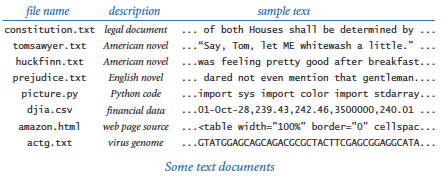Our interest is in finding efficient ways to search through the files using their content to characterize documents. One fruitful approach to this problem is to associate with each document a vector known as a sketch, which is an ultra-compact representation of its content. The basic idea is that the sketch should capture salient statistical features of the document, so that documents that are different have sketches that are "different" and documents that are similar have sketches that are "similar." These considerations lead to this API: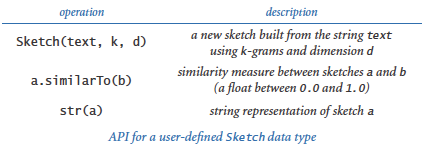The arguments of the constructor are a string and two integers that control the quality of the sketch. Clients can use `similarTo()` to determine the extent of similarity between two sketches on a scale of 0 (not similar) to 1 (similar).

### Computing sketches.

Computing a sketch of a document is the first challenge. Our first choice is to use a `Vector` to represent a document's sketch. Our implementation sketch.py uses a simple frequency count approach. In addition to the string, the constructor has two arguments, an integer k and a vector dimension d. It scans the document and examines all of the k-grams in the document — that is, the substrings of length k starting at each position. In its simplest form, the sketch is a vector that gives the relative frequency of occurrence of the k-grams in the string: an element for each possible k-gram giving the number of k-grams in the document that have that value.

### Hashing.

On many systems there are 128 different possible values for each character, so there are 128k possible k-grams, and the dimension d would have to be 128k for the simple scheme just described. This number is prohibitively large even for moderately large k. To ameliorate this problem, we use hashing, a fundamental operation that we considered earlier in this section to map an object to an integer. For any string `s`, `hash(s) % d` is an integer between 0 and `d`-1 that we can use as an index into an array to compute frequencies. The sketch that we use is the direction of the vector defined by frequencies of these values for all k-grams in the document (the unit vector with the same direction). The test client in sketch.py accepts `k` and `d` as command-line arguments, and computes and writes a sketch of the document that it reads from standard input. Try running it with standard input redirected to the file genome20.txt.

### Comparing sketches.

The second challenge is to compute a similarity measure between two sketches. One widely used similarity measure is known as the cosine similarity measure. Since our sketches are unit vectors with nonnegative coordinates, their dot product is a number between 0 and 1. The more similar the documents, the closer we expect this measure to be to 1. The `similarTo()` method in sketch.py uses that approach.

### Comparing all pairs.

The program comparedocuments.py is a simple and useful `Sketch` client that provides the information needed to solve the following problem: given a set of documents, find the two that are most similar. Since this specification is a bit subjective, the program writes the cosine similarity measure for all pairs of documents. The program accepts command-line arguments `k` and `d`, reads from standard input a list of file names, and writes a table showing the similarity measures of the files with those names. Try running it with standard input redirected to documents.txt, which contains the names of the files constitution.txt, tomsawyer.txt, huckfinn.txt, prejudice.txt, djia.csv, amazon.html, and actg.txt.

## Design-by-Contract

To conclude, we briefly discuss Python language mechanisms that enable you to verify assumptions about your program while it is running.

### Exceptions.

An exception is a disruptive event that occurs while a program is running, often to signal an error. The action taken is known as raising an exception (or error). We have already encountered exceptions raised by Python's standard modules in the course of learning to program: `IndexError` and `ZeroDivisionError` are typical examples. You can also raise your own exceptions. The simplest kind is an `Exception` that disrupts execution of the program and writes an error message:

 ```raise Exception('Error message here.') ```

It is good practice to use exceptions when they can be helpful to the client. For example, in vector.py, we should raise an exception in `__add__()` if the two `Vector`s to be added have different dimensions. To do so, we insert the following statement at the beginning of `__add__()`:

 ```if len(self) != len(other): raise Exception('vectors have different dimensions') ```

### Assertions.

An assertion is a boolean expression that you are affirming is `True` at that point in the program. If the expression is `False`, the program will raise an `AssertionError` at run time. Programmers uses assertions to detect bugs and gain confidence in the correctness of their programs. Assertions also serve to document the programmer's intent. For example, in counter.py, we might check that the counter is never negative by adding the following assertion as the last statement in `increment()`:

 ```assert self._count >= 0 ```

This statement would call attention to a negative count. You can also add an optional detail message, such as

 ```assert self._count >= 0, 'Negative count detected!' ```

to help identify the bug.

By default, assertions are enabled, but you can disable them from the command line by using the `-O` (that's a minus sign followed by an uppercase "oh") flag with the python command. (The `O` stands for "optimize.") Assertions are for debugging only; your program should not rely on assertions for normal operation since they may be disabled.

When using the design-by-contract model, the designer of a data type expresses a precondition (the condition that the client promises to satisfy when calling a method), a postcondition (the condition that the implementation promises to achieve when returning from a method), invariants (any condition that the implementation promises to satisfy while the method is executing), and side effects (any other change in state that the method could cause). During development, these conditions can be tested with assertions. Many programmers use assertions liberally to aid in debugging.

#### Q & A

Q. Why is the underscore convention not part of (and enforced by) Python?

A. Good question.

Q. Why all the leading underscores?

A. This is just one of many examples where a programming-language designer goes with a personal preference and we live with the result. Fortunately, most Python programs that you compose will be client programs, which do not directly call special methods or refer to private instance variables, so they will not need many leading underscores. The relatively few Python programmers who implement their own data types (that's you, now) need to follow the underscore conventions, but even those programmers are likely to be composing more client code than class implementations, so the underscores may not be so onerous in the long run.

Q. The `__mul__()` method in complexpolar.py is awkward because it create a `Complex` object (representing 0 + 0i) and then immediately changes its instance variables to the desired polar coordinates. Wouldn't the design be better if I could add a second constructor that takes the polar coordinates as arguments?

A. Yes, but we already have a constructor that takes the rectangular coordinates as arguments. A better design might be to have two ordinary functions (not methods) `createRect(x, y)` and `createPolar(r, theta)` in the API that create and return new objects. This design is perhaps better because it would provide the client with the capability to switch to polar coordinates. This example demonstrates that it is a good idea to think about more than one implementation when developing a data type. Of course, making such a change necessitates enhancing all existing implementations and clients of the API, so this thinking should happen as early in the design process as possible.

Q. How do I specify a tuple consisting of zero items or one item?

A. You can use `()` and `(1,)`, respectively. Without the comma in the second expression, Python would treat it as an arithmetic expression enclosed in parentheses.

Q. Do I really need to overload all six comparison methods if I want to make my data type comparable?

A. Yes. This is an example where the convention is to provide maximum flexibility to clients at the expense of extra code in implementations. Often, you can use symmetries to cut down on the actual amount of implementation code. Also, Python 3 supplies a few shortcuts. For example, if you define an `__eq__()` method for a data type but do not define an `__ne__()` method, then Python automatically provides an implementation that calls `__eq__()` and negates the result. However, Python 2 does not provide these shortcuts, so it is best not to rely upon them in your code.

Q. What is the range of integer values returned by the built-in `hash()` function?

A. Typically, Python uses a 64-bit integer, so the range is between -263 and 263-1. For cryptographic applications, you should use Python's `hashlib` module, which supports "secure" hash functions that support much larger ranges.

Q. Which Python operators cannot be overloaded?

A. In Python, you cannot overload

• The boolean operators `and`, `or`, and `not`.
• The `is` and `is not` operators, which test for object identity.
• The string format operator `%`, which can be applied only to strings.
• The assignment operator `=`.

#### Exercises

1. Create a data type `Location` for dealing with locations on Earth using spherical coordinates (latitude/longitude). Include methods to generate a random location on the surface of the Earth, parse a location "25.344 N, 63.5532 W", and compute the great circle distance between two locations.

2. Create a data type for a three-dimensional particle with position (rx, ry, rz), mass m, and velocity `(vx, vy, vz)`. Include a method to return its kinetic energy, which equals 1/2 m (vx2 + vy2 + vz2). Use the `Vector` data type, as defined in vector.py.

3. If you know your physics, develop an alternate implementation of the data type of the previous exercise based on using the momentum (px, py, pz) as an instance variable.

4. Develop an implementation of the `Histogram` class, as defined in histogram.py (from Section 3.2), that uses `Counter`, as defined in counter.py.

5. Compose an implementation of `__sub__()` for `Vector` (as defined in vector.py that subtracts two vectors.

Solution:

 ```def __sub__(self, other): return self + (other * -1.0) ```

Note that `__sub__()` calls `__add__()` and `__mul__()`. The advantage of such implementations is that they limit the amount of detailed code to check; the disadvantage is that they can be inefficient. In this case, `__add__()` and `__mul__()` both create new `Vector` objects, so copying the code for `__add__()` and replacing the minus sign with a plus sign is probably a better implementation.

6. Implement a data type `Vector2D` for two-dimensional vectors that has the same API as `Vector` (as defined in vector.py, except that the constructor takes two floats as arguments. Use two floats (instead of an array) for instance variables.

7. Implement the `Vector2D` data type of the previous exercise using one `Complex` object as the only instance variable.

8. Prove that the dot product of two two-dimensional unit-vectors is the cosine of the angle between them.

9. Implement a data type `Vector3D` for three-dimensional vectors that has the same API as `Vector`, except that the constructor takes three floats as arguments. Also, add a cross product method: the cross product of two vectors is another vector, defined by the equation

 a × b = c |a| |b| sin θ

where c is the unit normal vector perpendicular to both a and b, and θ is the angle between a and b. In Cartesian coordinates, the following equation defines the cross product:

 (a0, a1, a2) × (b0, b1, b2) = (a1 b2 - a2 b1, a2 b0 - a0 b2, a0 b1 - a1 b0)

The cross product arises in the definition of torque, angular momentum, and vector operator curl. Also, |a × b| is the area of the parallelogram with sides a and b.

10. Which modifications (if any) would you need to make `Vector` (see vector.py) work with `Complex` components (see complex.py from Section 3.2) or `Rational` components (see the "rational numbers" exercise in Section 3.2)?

11. Add code to charge.py (from Section 3.2) to make `Charge` objects comparable using the value of the charge to determine the order.

12. Compose a function `fibonacci()` that takes an integer argument `n` and computes the `n`th Fibonacci number. Use tuple packing and unpacking.

13. Revise the `gcd()` function in euclid.py (from Section 2.3) so that it takes two nonnegative integer arguments `p` and `q` and returns a tuple of integers (`d`, `a`, `b`) such that `d` is the greatest common divisor of `p` and `q`, and the coefficients `a` and `b` satisfy Bezout's identity: `d = a*p + b*q`. Use tuple packing and unpacking.

Solution: This algorithm is known as extended Euclid's algorithm:

 ```def gcd(p, q): if q == 0: return (p, 1, 0) (d, a, b) = gcd(q, p % q) return (d, b, a - (p // q) * b) ```
14. Discuss the advantages and disadvantages of Python's design in making the built-in type `bool` be a subclass of the built-in type `int`.

15. Add code to `Counter` (as defined in counter.py to raise a `ValueError` at run time if the client tries to create a `Counter` object using a negative value for `maxCount`.

16. Use exceptions to develop an implementation of `Rational` (see the "rational numbers" exercise in Section 3.2) that raises a `ValueException` at run time if the denominator is zero.

#### Data-Type Design Exercises

This group of exercises is intended to give you experience in developing data types. For each problem, design one or more APIs with API implementations, testing your design decisions by implementing typical client code. Some of the exercises require either knowledge of a particular domain or a search for information about it on the web.

1. Statistics. Develop a data type for maintaining statistics of a set of floats. Provide a method to add data points and methods that return the number of points, the mean, the standard deviation, and the variance. Develop two implementations: one whose instance values are the number of points, the sum of the values, and the sum of the squares of the values, and another that keeps an array containing all the points. For simplicity, you may take the maximum number of points in the constructor. Your first implementation is likely to be faster and use substantially less space, but is also likely to be susceptible to roundoff error.

2. Genome. Develop a data type to store the genome of an organism. Biologists often abstract the genome to a sequence of nucleotides (A, C, G, or T). The data type should support the methods `addCodon(c)` and `baseAt(i)`, as well as `isPotentialGene()` (see potentialgene.py from Section 3.1). Develop three implementations.

• Use a string as the only instance variable; implement `addCodon()` with string concatenation.
• Use an array of single-character strings as the only instance variable; implement `addCodon()` with the `+=` operator.
• Use a boolean array, encoding each base with two bits.
3. Time. Develop a data type for the time of day. Provide client methods that return the current hour, minute, and second, as well as a `__str__()` method. Develop two implementations: one that keeps the time as a single `int` value (number of seconds since midnight) and another that keeps three `int` values, one each for seconds, minutes, and hours.

4. Vector fields. Develop a data type for force vectors in two dimensions. Provide a constructor, a method to add two vectors, and an interesting test client.

5. Dates. Develop an API for dates (year, month, day). Include methods for comparing two dates chronologically, computing the number of days between two dates, determining the day of the week of a given date, and any other operations that you think a client might want. After you have designed your API, look at the Python's `datetime.date` data type.

6. Polynomials. Develop a data type for univariate polynomials with integer coefficients, such as x3 + 5x2 + 3x + 7. Include methods for standard operations on polynomials such as addition, subtraction, multiplication, degree, evaluation, composition, differentiation, definite integration, and testing equality.

7. Rational polynomials. Repeat the previous exercise, ensuring that the polynomial data type behaves correctly when provided coefficients of type `int`, `float`, `complex`, and `Fraction` (see the "rational numbers" exercise in Section 3.2).

#### Creative Exercises

1. Calendar. Develop `Appointment` and `Calendar` APIs that can be used to keep track of appointments (by day) in a calendar year. Your goal is to enable clients to schedule appointments that do not conflict and to report current appointments to clients. Use Python's `datetime` module.

2. Vector field. A vector field associates a vector with every point in a Euclidean space. Compose a version of potential.py (from Section 3.1) that takes as input a grid size n, computes the `Vector` value of the potential due to the point charges at each point in an n-by-n grid of equally spaced points, and draws the unit vector in the direction of the accumulated field at each point.

3. Sketching. Pick an interesting set of documents from the booksite (or use a collection of your own) and run comparedocuments.py with various command-line arguments, to learn about their effect on the computation.

4. Multimedia search. Develop sketching strategies for sound and pictures, and use them to discover interesting similarities among songs in the music library and photos in the photo album on your computer.

5. Data mining. Compose a recursive program that surfs the web, starting at a page given as the first command-line argument, and looks for pages that are similar to the page given as the second command-line argument, as follows. To process a name, open an input stream, do a `readAll()`, sketch it, and write the name if its distance to the target page is greater than the threshold value given as the third command-line argument. Then scan the page for all strings that contain the substring `http://` and (recursively) process pages with those names. Note: This program might read a very large number of pages!